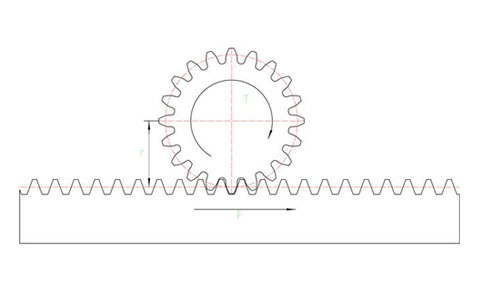Hello! Shenzhen Win-drive Automation Equipment Co., Ltd. welcomes you!
• Tik Tok• Weibo
• WeChat0755-82113520、13823217507(WeChat)Site map|Legal Notice|ChineseNews
Current location： Home News Industry news Gear rack driving force calculation formula and torque calculation formula
Gear rack driving force calculation formula and torque calculation formula

Rack and pinion is a very common transmission structure. Like screw drive, it converts rotary motion into linear motion. It is commonly used in laser cutting machines, engraving machines, large machining centers, elevators and other structures. When we calculate and select the rack and pinion, we need to calculate the maximum driving force and the maximum acceleration torque, so as to choose the appropriate motor and reducer.The following are some of the formulas needed to calculate the formula:

Acceleration: a=v÷t (v takes the maximum speed when the load is moving)

Driving force (horizontal): F=μ×m×g+m×a (μ is the safety factor)

Driving force (vertical): F=m×g+m×a (vertical transmission does not need to consider friction force)

Acceleration torque: T=F×r (r is the radius of the gear indexing circle)

Tangential force calculation:

Acceleration: a (m/s2))

Friction coefficient: μ=0.02 Safety factor: fs

Efficiency: n=95% (transmission efficiency of rack and pinion)

Friction force: f=μ*m*g+The small friction force exerted by the guide rail lubricating block on the guide rail (for the specific value, please refer to the sample)

Acceleration force: F acceleration = m*a

Total driving force during acceleration: horizontal application: F=(F acceleration+f)/n; vertical application: F=(lifting weight+F acceleration+f)/n

Considering the safety factor, F total=F*fs

Through the above formula, the maximum driving force and acceleration torque required by the load can be calculated, and it can be analyzed that: 1. The acceleration has a great influence on the driving force. The same load depends on the length of the acceleration time, and the required driving force will be different. The difference is very large; 2. When vertical transmission, it is necessary to overcome all gravity, so it is necessary to choose a motor with a brake; 3. The radius of the gear index circle will affect the torque, so on the premise that the strength of the gear can be satisfied, reduce as much as possible Gear radius, which reduces the torque requirements of the motor.

Tel: 0755-82113520, 13823217507
E-mail: sales@sz -windrive. com
URL: www.sz-windrive.com com
Address: room 708, MaoYuan building, No. 9, Songyuan Road, Guiyuan street, Luohu District, ShenzhenWeChat